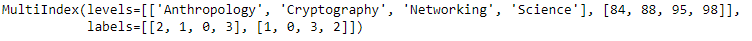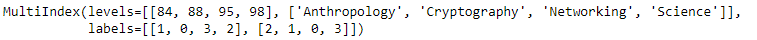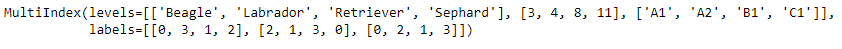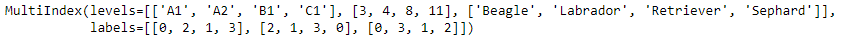Open in App
Not now

# Python | Pandas MultiIndex.swaplevel()

• Last Updated : 24 Dec, 2018

Python is a great language for doing data analysis, primarily because of the fantastic ecosystem of data-centric python packages. Pandas is one of those packages and makes importing and analyzing data much easier.

Pandas` MultiIndex.swaplevel()` function is used to swap levels of the MultiIndex. It swap level i with level j. Calling this method does not change the ordering of the values.

Syntax: MultiIndex.swaplevel(i=-2, j=-1)

Parameters :
i : First level of index to be swapped. Can pass level name as string. Type of parameters can be mixed.
j : Second level of index to be swapped. Can pass level name as string. Type of parameters can be mixed.

Returns : A new MultiIndex

Example #1: Use `MultiIndex.swaplevel()` function to swap the 0th level with the 1st level of the MultiIndex.

 `# importing pandas as pd ` `import` `pandas as pd ` ` `  `# Create the MultiIndex ` `midx ``=` `pd.MultiIndex.from_arrays([[``'Networking'``, ``'Cryptography'``,  ` `                                     ``'Anthropology'``, ``'Science'``],  ` `                                             ``[``88``, ``84``, ``98``, ``95``]]) ` ` `  `# Print the MultiIndex ` `print``(midx) `

Output :Now let’s swap the 0th level with the 1st level of the MultiIndex.

 `# swap the levels ` `midx.swaplevel(``0``, ``1``) `

Output :As we can see in the output, the function has swapped the 0th level with the 1st level of the MultiIndex.

Example #2: Use `MultiIndex.swaplevel()` function to swap the 0th level with the 1st level of the MultiIndex.

 `# importing pandas as pd ` `import` `pandas as pd ` ` `  `# Create the MultiIndex ` `midx ``=` `pd.MultiIndex.from_arrays([[``'Beagle'``, ``'Sephard'``, ``'Labrador'``, ``'Retriever'``], ` `                                       ``[``8``, ``4``, ``11``, ``3``], [``'A1'``, ``'B1'``, ``'A2'``, ``'C1'``]]) ` ` `  `# Print the MultiIndex ` `print``(midx) `

Output :Now let’s swap the 0th level with the 2nd level of the MultiIndex.

 `# swap the levels ` `midx.swaplevel(``0``, ``2``) `

Output :As we can see in the output, the function has swapped the 0th level with the 2nd level of the MultiIndex.

My Personal Notes arrow_drop_up
Related Articles